MPA HOMEPAGE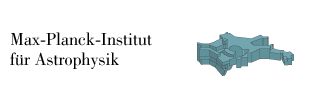Current Research Highlight :: August 2009 all highlights## Mathematics of digital senses: Information Field Theory for signal recognition

The correct interpretation of signals through our senses is not only an essential problem of living creatures, but also of fundamental scientific relevance. Scientists at the Max-Planck-Institute for Astrophysics have shown that mathematical methods from particle physics can be used for developing image reconstruction techniques. These yield optimal results even for incomplete, defective, and distorted data. Information Field Theory, which is used to develop such image reconstruction techniques, provides us with algorithms, i.e. mathematical instructions, for computing complicated perception processes in engineering and science, such as in cosmology.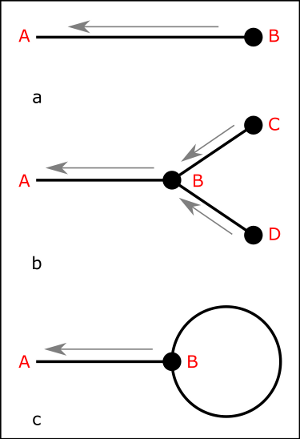Fig. 1: The Feynman diagrams consist of points and lines. Points at the end of lines are so-called information sources, i.e. the information about a point in space which is contained in the data (see diagram (a)). Points embedded in several lines stand for data processing steps that combine different pieces of information (see diagram (b)). The lines describe the propagation of information: What does an information source at position B tell me about the signal at position A? Each of the possible diagrams describes a mathematical instruction, an algorithm, which has to be applied to the data. The sum of the results of the respective diagrams yields the desired answer to our problem, e.g. the mean signal given data and previous knowledge.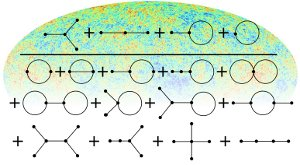Fig. 2: Diagrams to determine the deviation of the CMB temperature fluctuations from a normal distribution, which is caused by inflation. The values of the CMB temperature (here encoded in colour) have to pass through the depicted diagrams. The temperature values of every point in the sky are put into the end points of every diagram, and are then processed according to the computational prescription given by the diagram. The given combination of the diagrams yields the strength of the statistical deviation, and thus a measurement of a signature of inflation. The numerator of this formula, consisting of four diagrams, is already well-known in a non-diagrammatic form in the technical literature. The denominator, however, which encodes the measurement uncertainty of the method, has only been known in a much cruder form before.Human senses like hearing, sight, and touch allow our brain to create a detailed image of our surroundings, even though the human senses are by no means perfect and our field of vision is often limited. Our brain accomplishes this by using its knowledge about the configuration of our surroundings.

Artificial "senses" like cameras, microphones or astronomical telescopes are also not perfect and thus need sophisticated interpretation and the use of additional information. For instance, cosmologists want to precisely measure the distribution of matter in the Universe, but they have the problem that 85 per cent of the matter is invisible. We see the visible matter in form of galaxies, but their light is not always caught by our telescopes. Therefore, the galaxy data only yield an incomplete and noisy image of the cosmic matter distribution. In this example, the signal which we want to reconstruct from the data is the matter distribution.

Since there are a huge number of possible matter distributions, the question is which of them is the correct signal. Unfortunately, the same data could have been created by infinitely many different signals. However, not all of these possibilities are equally plausible. It is, for instance, not very likely that the cosmic matter distribution in the region obscured by our galaxy is completely different from the distribution in the other regions, just because we happen to not be able to observe this region very well. After all, we wouldn't think that a person has three legs just because we cannot see his legs at the time we look at him. That is, we can a priori assign a small probability to exotic signal configurations.

With this in mind, we can ask questions like: Which signal is the most plausible one, given the data and previous knowledge? How large is the uncertainty in this estimate of the signal?

The scientists at the Max-Planck-Institute for Astrophysics have shown that such questions can be formulated as a statistical field theory, the so-called Information Field Theory. The latter is very complex, but thanks to particle physics there exist powerful mathematical tools for handling it. We can, for instance, obtain approximate solutions to our problems by using so-called Feynman diagrams (see Fig. 1). These diagrams are graphical descriptions of the data processing steps.

Not all of the resulting algorithms are entirely new. The simplest of the diagrams (Fig. 1a) corresponds to the Wiener filter, which has been successfully used for the last 60 years. More complex diagrams (Figs 1b&c) can be constructed from the single steps of the Wiener filter, and they allow us to tackle signal recognition problems which are not optimally treated by the Wiener filter. Here, Information Field Theory yields optimal algorithms tailored to the respective signal recognition problem.

The first applications of this new methodology will be in the field of cosmology. Optimal methods for cosmic cartography using galaxy observations, which are supposed to improve existing linear methods (see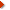Research Highlights October 2008), have already been developed.

We can also obtain more accurate insights about the early Universe shortly after the Big Bang using Information Field Theory. We obtain the earliest possible map of the Universe from the cosmic microwave background (CMB). The latter is an image of the hot gas permeating the Universe 380,000 years after the Big Bang. It contains information about the first fractions of a second of our Universe, when, during the so-called 'Inflationary Epoch', space itself was practically exploding.

The CMB can be measured by satellites like the recently launched Planck Surveyor (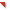Planck News). But Planck will also measure unwanted signals, which makes it difficult to directly interpret the data. For example, radiation from our own galaxy blocks a part of the CMB sky, and the detectors of the satellites produce unwanted noise in the data. In order to detect the subtle signatures of inflation in the temperature fluctuations in spite of these additional noise signals, Information Field Theory has been used to derive improved analysis techniques (Fig. 2).

The utility of Information Field Theory is by no means restricted to cosmology. Imaging techniques in medicine, geology, and material science could possibly benefit from the theory. Should this happen, it would be one further example of an unexpected spin-off from fundamental research: Methods from mathematical physics, which have been developed for computing very abstract processes in particle physics would enter medical practices and engineering companies in the form of software.

Torsten Enßlin, Mona Frommert

#### Publication

Torsten A. Enßlin, Mona Frommert, Francisco S. Kitaura, "Information field theory for cosmological perturbation reconstruction and non-linear signal analysis", 2008, submittedarXiv:0806.3474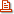print version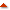Top© 2003—2022, Max-Planck-Gesellschaft, München last modified: 2009-7-23